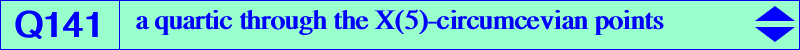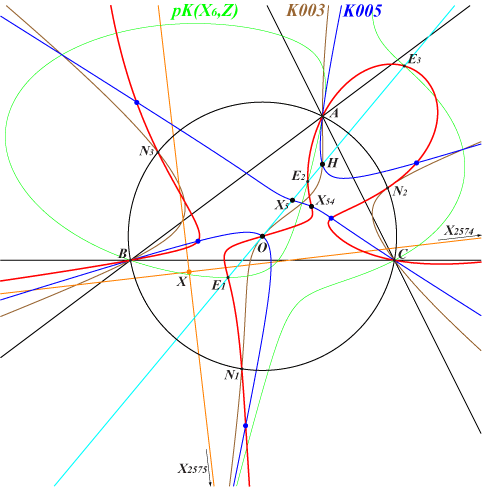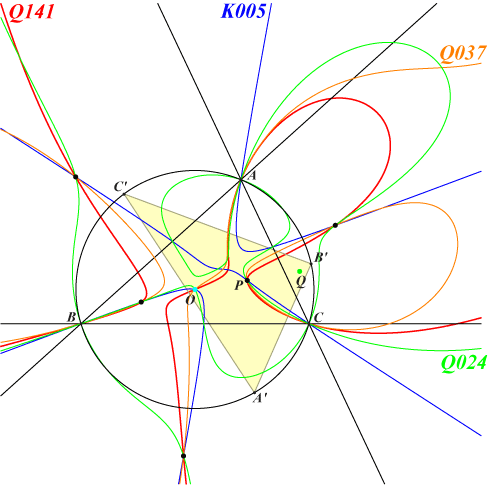too complicated to be written here. Click on the link to download a text file.X(3), X(54), X(2574), X(2575), X(6759), X(13557) vertices of the CircumNormal triangle four X3-OAP points, see Table 53 X(5)-circumcevian points (blue points) foci of the inconic with center X(140) other points belowQ141 is a circular quartic with singular focus X(186). It meets the line at infinity again at the same points as the Jerabek hyperbola. The tangents at these points meet at X on the lines {3,1568}, {113,2071}, {125,539}, {140,389}, etc, with SEARCH = 3.83823890657063. X is now X(14156) in ETC (2017-08-26). Q141 passes through X(3), a point of inflexion with tangent passing through X(49). The three remaining intersections E1, E2, E3 with the Euler line lie on the cubic pK(X6, Z) with Z on the lines {4,54}, {24,1192}, {30,110}, {74,186}, {112,1971}, {113,3153}, {154,378}, {156,382}, etc, with SEARCH = -5.64972330061297. Z is now X(14157) in ETC (2017-08-26). The tangents at A, B, C pass through X(3). Q141 meets the Napoleon cubic K005 at A, B, C, X(3), X(54) and seven other points which are the X(5)-circumcevian points. These points also lie on Q024 and Q037.The X(5)-circumcevian points Consider a point P with circumcevian triangle A'B'C'. Its circumcenter is obviously O = X(3) and let us denote by Q, N its orthocenter and its nine-point center respectively. P is said to be a X(5)-circumcevian point if P and N coincide i.e. P is the nine-point center of its circumcevian triangle. There are seven such points but they are not necessarily all real. In the opposite figure, five only are real. *** Recall that the locus of P such that P, N, X(3) [resp. X(5)] are collinear is Q037 together with (O) [resp. Q024 together with the line at infinity]. More generally, the locus of P such that P, N and a fixed point M are collinear is a tricircular circum-septic passing through M and the X(5)-circumcevian points.